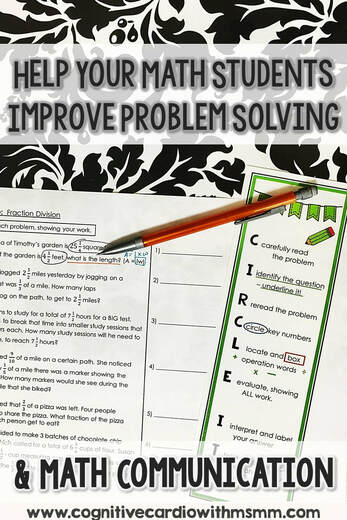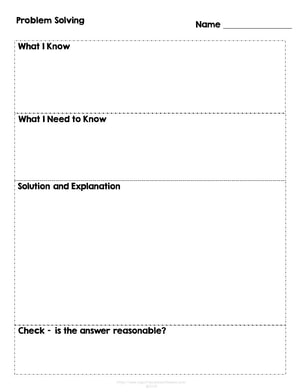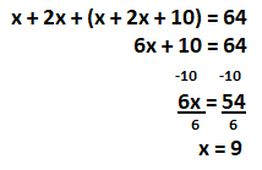# Help Middle School Math Students Improve Problem Solving Skills

Ways to Improve Problem Solving Skills and Math CommunicationDo Your Students Struggle with Word Problems?
Do your middle school math students struggle with problem solving? Do they get to the end of the word problem and then guess at the operation they need to choose (maybe not realizing that there are multiple operations)? You probably see this with some of your students, while other students do very well with problem solving. What methods have you found to help those who struggle? What methods can you use to help each student at his or her current level?

I’ve used many strategies over the years, to help students sort out how to make sense of word problems and how to approach them. These methods didn’t have a specific name at the time (like close reading or talking to the text), but some would fit into these categories.Click to download free recording sheet.

Prompts to Help Focus
One of the methods I found to be most helpful for my math students was having them write responses to specific prompts before they attempted to solve a word problem. The prompts are general and applicable to any problem:

• “What I know…because,” from the problem
• “What I know…because,” from background information and
• “What I need to know….,” or what the problem is asking

We started using this framework many years ago, when writing in math/open-ended questions was new on the standardized test scene (new in my state any way:-). Every couple of days, we did sample problems that incorporated various strategies to solve problems – make a simpler problem, make a table, make an organized list, write an equation, etc. And as we practiced, the students became excellent at communicating what they understood about the information provided in the problem, as well as what they needed to figure out and how they did so.

For the “what I know…because” part, students identify the information from the problem that’s important for solving. We’d typically underline or highlight important information and cross out extra information. Instead of just highlighting/underlining, students also wrote the information, putting it into their own words as much as possible. Writing the information helps solidify it in their minds, and if they reword it or add detail to clarify the meaning, they understand it a bit better.

For “what I need to know,” students highlight/underline what the question is asking and then wrote it in their own words.

For example, with a problem like this:
Steve runs every other day and trains with weights every 3rd day. If he does both on Monday, how many times will he do both on the same day during the next 2 weeks?

Students might write:
What I know:

• Runs every 2 days. I know this because every other day means the same things as every 2 days.
• Weights every 3rd day – the problem states this information.
• Runs and uses weights on Monday – the problem states that he does both activities on Monday.

What I need to know:
I need to figure out how many times will Steve run and use weights on the same day, during the next 14 days – I know that 2 weeks is the same as 14 days.

After students completed these written parts, we’d discuss what they identified as what they knew and what they needed to know,  before getting started with the solving. Then students would solve on their own and write a paragraph to explain exactly what they did to solve the problem.

Solution Explanation Example:
To solve this problem, I decided to make a table to find how many days Steve will do both activities. Since I want to know how many times this happened in 2 weeks, I made the table 2 rows of 7, and I labeled the days of the week at the top of the table, starting with Monday. In the first square of the table, I wrote an R and a W, since Steve did both on Monday. Then I wrote an R in every other square, and I wrote a W in every 3rd square. When I was finished, I counted how many squares had both R and W in them. There were 3 days total (including the Monday he started), so the answer is: Steve will do both activities on the same day 3 different times in 2 weeks.

Once students finished solving and writing their paragraphs, several of them would read their paragraphs to the class, giving students the opportunity to see if they could follow their peers’ explanations, compare the explanations to their own to see how similar they were, and learn/consider a new method if a student had solved a different way.

While this process did take a while, it was SO worth it. It really helped students break down the problems, become more in tune with how they were solving, and resulted in less “random” use of operations/solving methods. It also greatly improved their math communication abilities.

These days, with shorter math classes:-(, and therefore less time to write, I’ve consolidated the “what I know” and “what I need to know” into ‘Find out,’ so it encompasses both the important info the question that needs to be answered. Where I used to have several students read their examples with the class, I now have students do a quick “pair-share” after the first stages, and then share a couple of the final explanations with the entire class. It still takes a good chunk of time, but I believe that time is made up with fewer struggles as we move through the year.

A few tips to help students as they read the problem:

• Underline important information
• Cross out information that isn’t needed
• Read carefully to find numbers written as words and write the # above the word
• Underline or highlight words that indicate operations and add the operation symbol nearby
• When writing, write the information with fewer words, so key info doesn’t get “lost”

I created some handy bookmarks that I give my students, to help them remember some of these ideas:-)Here’s another example, in case you want to keep reading!
Manny spent 64 minutes on 3 different subway trains. The first train ride was twice as long as the second. The third train ride was 10 minutes longer than the other two combined. He arrived at his destination at 4:00 in the afternoon. How long was each train ride?

What I know:
64 minutes
3 different trains

Train 1 = 2 x as long as train 2
(change words into numbers – sub words with symbols – x instead of times)
Train 3  = 10 min longer than train 1 + train 2

What I need to know:
I need to find the length of each train ride.

Solution Explanation:
I decided to write an equation and use x as the variable to represent the shortest ride.
I know that all 3 rides add up to 64, so 64 is one side of the equation.
I think that train 2 is the shortest time, because train 1 is 2  times as long, so train 2 is  x and train 1 is  2x.
Train 3 is 10 more than the other trains combined, so train 3 is  x+ 2x + 10.
This gives me the equation  x + 2x + (x + 2x + 10) = 64.

I solve by combining all the like terms, which gives me
6x + 10 = 64. Then I subtract 10 from both sides to isolate the variable and that gives me 6x = 54. Next I divide both sides by 6 and get x = 9.

I have to  figure out the other 2 trains based on train 2 = 9.
If train  2 = 9, then train 1 (2 (9)) = 18 and train 3 (9 + 2(9) + 10 ) = 37.
9 + 18 + 37 = 64, which is the total number of minutes Manny spend on the trains.

## EllieWelcome to Cognitive Cardio Math! I’m Ellie, a wife, mom, grandma, and dog ‘mom,’ and I’ve spent just about my whole life in school! With nearly 30 years in education, I’ve taught:

• All subject areas in 4th and 5th grades
• Math, ELA, and science in 6th grade (middle school)

I’ve been creating resources for teachers since 2012 and have worked in the elearning industry for about five years as well!